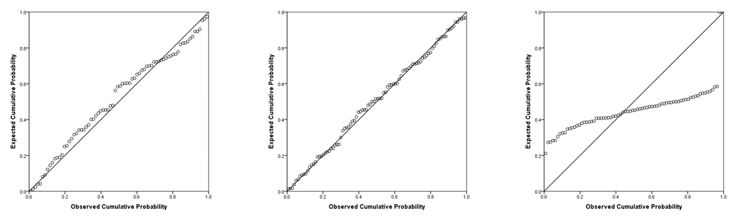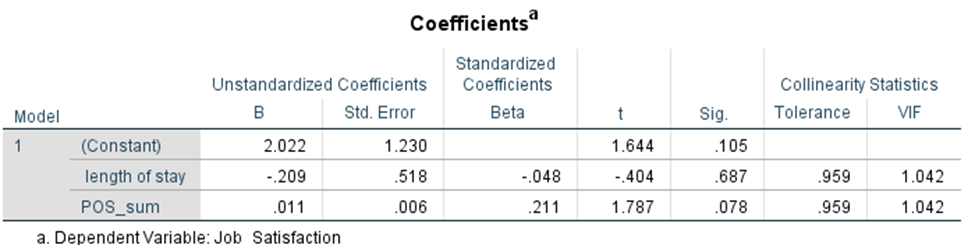# Testing Assumptions of Linear Regression in SPSS

Posted October 11, 2017

You have finally defended your proposal, found your participants, and collected your data. You have your rows of shiny, newly collected data all set up in SPSS, and you know you need to run a regression. If you have read our blog on data cleaning and management in SPSS, you are ready to get started! But you cannot just run off and interpret the results of the regression willy-nilly. First, you need to check the assumptions of normality, linearity, homoscedasticity, and absence of multicollinearity. Homosced-what? Collinearity? Don’t worry, we will break it down step by step.

We will start with normality. In order to make valid inferences from your regression, the residuals of the regression should follow a normal distribution. The residuals are simply the error terms, or the differences between the observed value of the dependent variable and the predicted value. If we examine a normal Predicted Probability (P-P) plot, we can determine if the residuals are normally distributed. If they are, they will conform to the diagonal normality line indicated in the plot. We will show what this looks like a little bit later.

Homoscedasticity refers to whether these residuals are equally distributed, or whether they tend to bunch together at some values, and at other values, spread far apart. In the context of t-tests and ANOVAs, you may hear this same concept referred to as equality of variances or homogeneity of variances. Your data is homoscedastic if it looks somewhat like a shotgun blast of randomly distributed data. The opposite of homoscedasticity is heteroscedasticity, where you might find a cone or fan shape in your data. You check this assumption by plotting the predicted values and residuals on a scatterplot, which we will show you how to do at the end of this blog.

Linearity means that the predictor variables in the regression have a straight-line relationship with the outcome variable. If your residuals are normally distributed and homoscedastic, you do not have to worry about linearity.

Multicollinearity refers to when your predictor variables are highly correlated with each other. This is an issue, as your regression model will not be able to accurately associate variance in your outcome variable with the correct predictor variable, leading to muddled results and incorrect inferences. Keep in mind that this assumption is only relevant for a multiple linear regression, which has multiple predictor variables. If you are performing a simple linear regression (one predictor), you can skip this assumption.

You can check multicollinearity two ways: correlation coefficients and variance inflation factor (VIF) values. To check it using correlation coefficients, simply throw all your predictor variables into a correlation matrix and look for coefficients with magnitudes of .80 or higher. If your predictors are multicollinear, they will be strongly correlated. However, an easier way to check is using VIF values, which we will show how to generate below. You want these values to be below 10.00, and best case would be if these values were below 5.00.

To fully check the assumptions of the regression using a normal P-P plot, a scatterplot of the residuals, and VIF values, bring up your data in SPSS and select Analyze –> Regression –> Linear. Set up your regression as if you were going to run it by putting your outcome (dependent) variable and predictor (independent) variables in the appropriate boxes.But don’t click OK yet! Click the Statistics button at the top right of your linear regression window. Estimates and model fit should automatically be checked. Now, click on collinearity diagnostics and hit continue.The next box to click on would be Plots. You want to put your predicted values (*ZPRED) in the X box, and your residual values (*ZRESID) in the Y box. Also make sure that normal probability plot is checked, and then hit continue.Now you are ready to hit OK! You will get your normal regression output, but you will see a few new tables and columns, as well as two new figures. First, you will want to scroll all the way down to the normal P-P plot. You will see a diagonal line and a bunch of little circles. Ideally, your plot will look like the two leftmost figures below. If your data is not normal, the little circles will not follow the normality line, such as in the figure to the right. Sometimes, there is a little bit of deviation, such as the figure all the way to the left. That is still ok; you can assume normality as long as there are no drastic deviations.The next assumption to check is homoscedasticity. The scatterplot of the residuals will appear right below the normal P-P plot in your output. Ideally, you will get a plot that looks something like the plot below. The data looks like you shot it out of a shotgun—it does not have an obvious pattern, there are points equally distributed above and below zero on the X axis, and to the left and right of zero on the Y axis.If your data is not homoscedastic, it might look something like the plot below. You have a very tight distribution to the left of the plot, and a very wide distribution to the right of the plot. If you were to draw a line around your data, it would look like a cone.Finally, you want to check absence of multicollinearity using VIF values. Scroll up to your Coefficients table. All the way at the right end of the table, you will find your VIF values. Each value is below 10, indicating that the assumption is met.You will want to report the results of your assumption checking in your results chapter, although school guidelines and committee preferences will ultimately determine how much detail you share. It is always best to err on the side of caution, and include the APA-formatted figures as well as your VIF values in your results chapter. After testing these assumptions, you will be ready to interpret your regression!

Intellectus allows you to conduct and interpret your analysis in minutes. Assumptions are pre-loaded, and output is provided in APA style complete with tables and figures. Click ‘Try Now’ below to create a free account, and get started analyzing your data now!

Shares